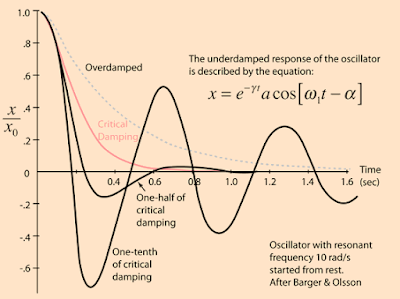## Pages

### 4.1 Damping, Forced Oscillation and Resonance

In this section we'll look at the the topics mention in the title briefly,

If an oscillator is displaced and then released it will begin to vibrate. If no more external forces are applied to the system it is a free oscillator. In absense of dissipative forces, the total energy of free oscillator will remain constant and the oscillations will continue forever. (e.g. ideal simple pendulum)

In real world, real oscillations tend to die out with time. Damping refers to the reduction of the amplitude of an oscillating system due to loss of energy caused by dissipative forces such as friction and viscosity. Damping is classified into the following,
• Light Damping: When a system is displace and it oscillates with gradual decreasing of the amplitude, such damping is called the light damping (as represented by $1/10^{th}$ critical damping in the plot). e.g. real world pendulum.
• Critical Damping: When the system is displaced and the system returns to the rest at its equilibrium position in the shortest possible time without oscillating, such damping is called the critical damping. (e.g. shock absorber in the car)
• Heavy Damping: When the system is displaced and it takes a long time to return to its equilibrium position, such damping is called the heavy damping.(e.g. anti slamming doors devices.)
A graphical representation(Amplitude vs time) of the damping types is shown below,http://hyperphysics.phy-astr.gsu.edu/hbase/oscda.html

A beautiful video showing the light damping, critical damping and heavy damping:

Forced Oscillation: As we know that any oscillating system in the real world, the oscillation finally comes to halt due to damping effect. So in order to sustain the oscillation we have to apply the periodic force. The oscillation in which a force is continually or repeatedly applied to keep the oscillation going, it is called a forced oscillator. e.g. To sustain a childs swinging we apply a periodic force.

When a system is oscillating it oscillates at its natural frequency ($f_{0}$). The (varying) periodic driving force also has its own frequency associated with it. Now if we vary the driving frequency ($f_{d}$) slowly we see a significant maximum amplitude at certain frequency($f_{d}=f_{0}$). This phenomenon is called a resonance and the frequency at which it happens is called the resonance frequency.

A wonderful demostration of the resonance effect is shown in the video below,

The characteristics plot of the amplitude vs driving frequency curve is shown below,
As we can see from the plot on the above diagram that the height and the shape of the plot depends upon the extent of damping. If there is no damping theoretically the amplitude would be infinite. If there is little damping the amplitude of the resonance is comparatively large compared to that of the critical and the heavy damping.

Resonance can be sometimes useful(in tuning a radio communication) and sometimes not so useful as shown in the video below.

Next section we'll talk about waves.
ref: damping